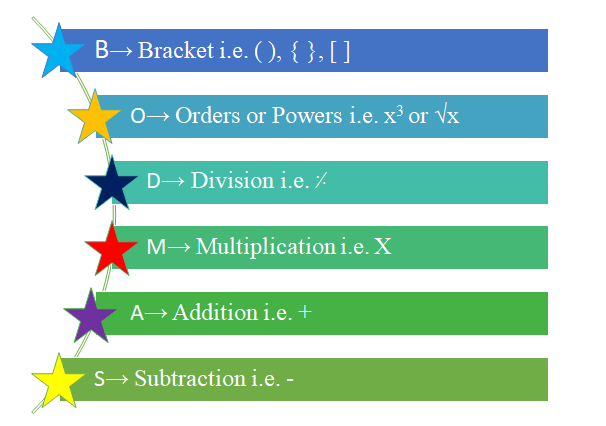# BODMAS Rule

In mathematics, while solving the arithmetic equations i.e. mathematical operations on numbers we have to obey some rules and then only we will get the correct answer. Its look like a simple but not like that, we have to solve the numerical carefully according to BODMAS.

BODMAS is the acronym which is having meaning bracket, orders or powers, division or multiplication, addition or subtraction. It gives the order of operations we have to do while solving the problems.

Hence, first we have to solve brackets, then we have to solve orders i.e. power or root terms then we have to solve division or multiplication as which comes first from left side of the equation and finally we have to do addition or subtraction.For example:

1) 26 ⸓ 2 x 6 – 42 + (7 + 8) =?

Now, according to BODMAS first we solve bracket.

= 26 ⸓ 2 x 6 – 42 + 15 Bracket is solved (7 + 8) = 15

= 26 ⸓ 2 x 6 – 16 + 15                      Order means power is solved 42 = 16

= 13 x 6 – 16 + 15                             Division is solved 26 ⸓ 2

= 78 – 16 + 15                                    Multiplication is solved 13 x 6 = 78

= 93 – 16Addition is solved 78 + 15 = 93

= 77                                                         Subtraction from left is first 93 – 16 = 77

Thus, 26 ⸓ 2 x 6 – 42 + (7 + 8) = 77

2) 5 + 4 – 12 ⸓ 3(7 – 5) =?

Now, according to BODMAS rule we solve the problem as follows.

= 5 + 4 -12 ⸓ 3 x 2                                     Bracket is solved 7 – 5 = 2

= 5 + 4 – 4 x 2                                           Division is solved 12 ⸓ 3 = 4

= 5 + 4 – 8                                                  Multiplication is solved 4 x 2 = 8

= 9 – 8                                                         Addition is solved 5 + 4 = 9

= 1                                                                Subtraction is solved 9 – 8 = 1

Thus, 5 + 4 – 12 ⸓ 3(7 – 5) = 1

3) 6 + [2 + 8 – 9 {4 x 2 – 3 + (2 x 4 ⸓4)}] =?

Now, according to BODMAS rule we solve this problem.

We have to note that, the order of solving bracket is as follows.

1→ simple bracket i.e. ( )

2→ curly bracket i.e. { }

3→ square bracket i.e. [ ]

Now by using above method, we solve completely.

= 6 + [2 + 8 – 9 {4 x 2 – 3 + (2 x 1)}]             Division in ( ) is solved 4 ⸓ 4 = 1

= 6 + [2 + 8 – 9 {4 x 2 – 3 + 2}]   Multiplication in ( ) is solved 2 x 1 = 2

= 6 + [2 + 8 – 9 {8 – 3 + 2}]        Multiplication in { } is solved 4 x 2 = 8

= 6 + [2 + 8 – 9{10– 3}]                                           Addition in { } is solved 8 + 2 = 10

= 6 + [2 + 8 – 9{7}]                                                  Subtraction in { } is solved 10 – 3 = 7

= 6 + [2 + 8 – 63]                         Multiplication in [ ] is solved 9{7} = 63

= 6+ [10 – 63]                               Addition in [ ] is solved 2 + 8 = 10

= 6 + [-53]                                     Subtraction in [ ] is solved 10 – 63= -53

= 6 – 53

= -47 Subtraction is solved 6 – 53= -47

Thus, 6 + [2 + 8 – 9 {4 x 2 – 3 + (2 x 4 ⸓4)}] = -47

Also See: PEMDAS or BODMAS MCQ with Answers

Updated: November 3, 2022 — 2:45 pm